Note: The other languages of the website are Google-translated. Back to English

## Excel Formula: Add months to date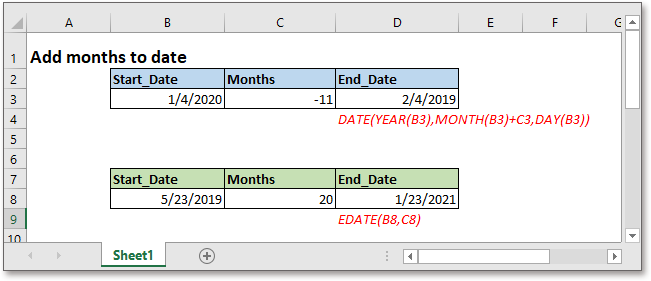in this tutorial, it introduces formulas to add months to a given date in Excel, and explains how the formulas work.

### Formula 1

Generic formula:

 DATE(YEAR(date),MONTH(date))+months,DAY(date)))

Arguments

 Date: the date you want to add months to. Months: a whole number which represents the number of months you want to add. It adds months to dates while the argument months is a positive value, and it subtracts months from the given date while the argument months is negative.

Return value

Return a value in date format.

How this formula work

Take an instance, to add months to the date in cell B3, and the number of months is placed in cell C3, please use the formula as below:

 =DATE(YEAR(B3),MONTH(B3)+C3,DAY(B3))

Press Enter key to get the result.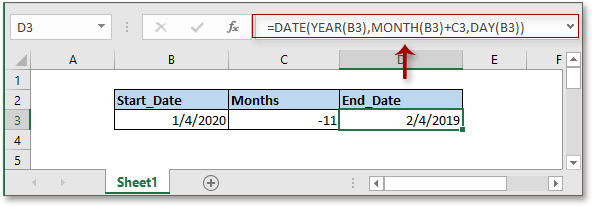Explanation

YEAR, MONTH and DAY functions separately get the year, month and day as relative integers from the given date.

Date function is used to create a date based on the given year, month, and day.

### Formula 2

Generic formula:

 EDATE(Sart_date,months)

Arguments

 Start_date: the date you want to add months to. Months: a whole number which represents the number of months you want to add. It adds months to dates while the argument months is a positive value, and it subtracts months from the given date while the argument months is negative.

Return value

Return a seriealn number value.

How this formula work

For example, to add months to the date in cell B8, and the number of months is placed in cell C8, please use the formula as below:

 =EDATE(B8,C8)

Or directly use the number in the formula

 =EDATE(B8,20)

Press Enter key to get the date in serial number format.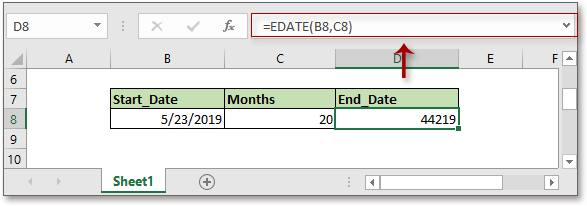Explanation

There are 24 hours a day, and one hour is 1/24 day.

Remark

If you want to subtract the hours from time, please use below formula

 MOD(Start_time-hours/24,1)

For example, there is a time in cell B18, the number of hours you want to subtract is displayed in cell C18, please use below formula:

=MOD(B18-C18/24,1)

Or

=MOD(B18-12/24,1)

Press Enter key then the result is shown.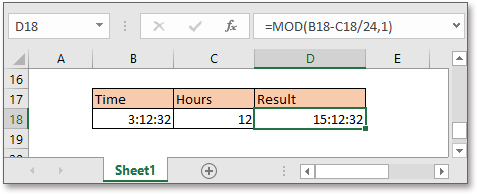Then right click at the serial number, choose Format Cells from the context menu, then in the pipping Format Cells dialog, choose Date in Category list under Number tab, and select one type of date formats you want the result displayed.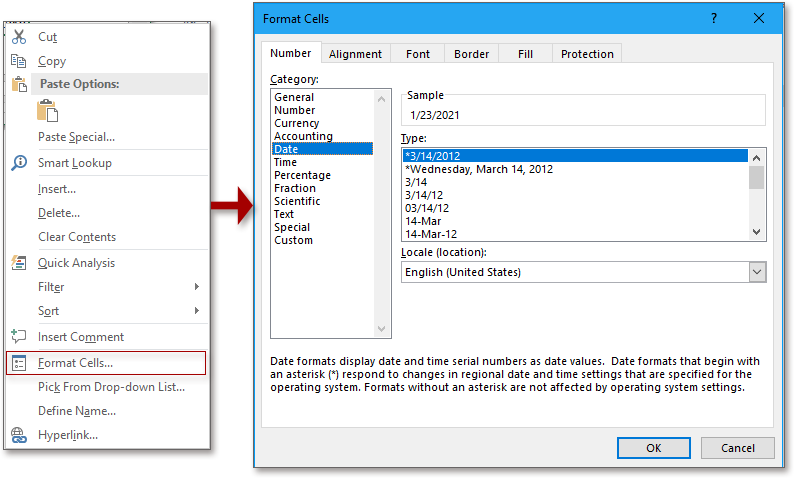Click OK, then the serial number is displayed in date format.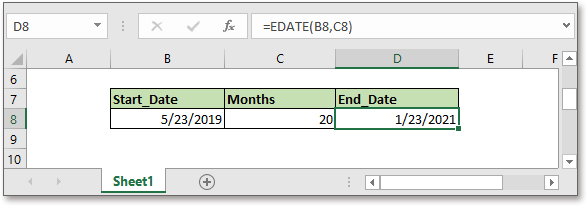Note:

Excel stores dates as sequential serial numbers so that they can be used in calculations. By default, January 1, 1900 is the serial number 1. In this case, serial number 44219 means the day is 44219 days after January 1, 1900, and it is 1/23/2021.

Explanation

The EDATE function is used to add months to a date, for more details about this function, please go to this article Excel EDATE Function.

### Sample File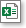Click to download sample file

#### Relative Formulas

• Add Hours Minutes Seconds To Time
Supposing there is a list of time values in Excel, now you want to add 3 hours 45 minutes and 20 seconds to the time values, Excel provides two formulas to help you.
• Extract Or Get Date Only From The Datetime In Excel
To extract only date from a list of datetime cells in Excel worksheet, the INT, TRUNC and DATE functions can help you to deal with this job quickly and easily.
• Add Minutes To Time
This tutorial provides formulas and explains how to add minutes to time in Excel.
• Get Work Hours Between Two Dates In Excel
The NETWORKDAYS function in Excel can help you to get the net workdays between two dates, and then multiply the number of working hours per workday to get the total work hours.

### The Best Office Productivity Tools

#### Kutools for Excel - Helps You To Stand Out From Crowd

Would you like to complete your daily work quickly and perfectly? Kutools for Excel brings 300 powerful advanced features (Combine workbooks, sum by color, split cell contents, convert date, and so on...) and save 80% time for you.

• Designed for 1500 work scenarios, helps you solve 80% Excel problems.
• Reduce thousands of keyboard and mouse clicks every day, relieve your tired eyes and hands.
• Become an Excel expert in 3 minutes. No longer need to remember any painful formulas and VBA codes.
• 30-day unlimited free trial. 60-day money back guarantee. Free upgrade and support for 2 years.#### Office Tab - Enable Tabbed Reading and Editing in Microsoft Office (include Excel)

• One second to switch between dozens of open documents!
• Reduce hundreds of mouse clicks for you every day, say goodbye to mouse hand.
• Increases your productivity by 50% when viewing and editing multiple documents.
• Brings Efficient Tabs to Office (include Excel), Just Like Chrome, Firefox, And New Internet Explorer.